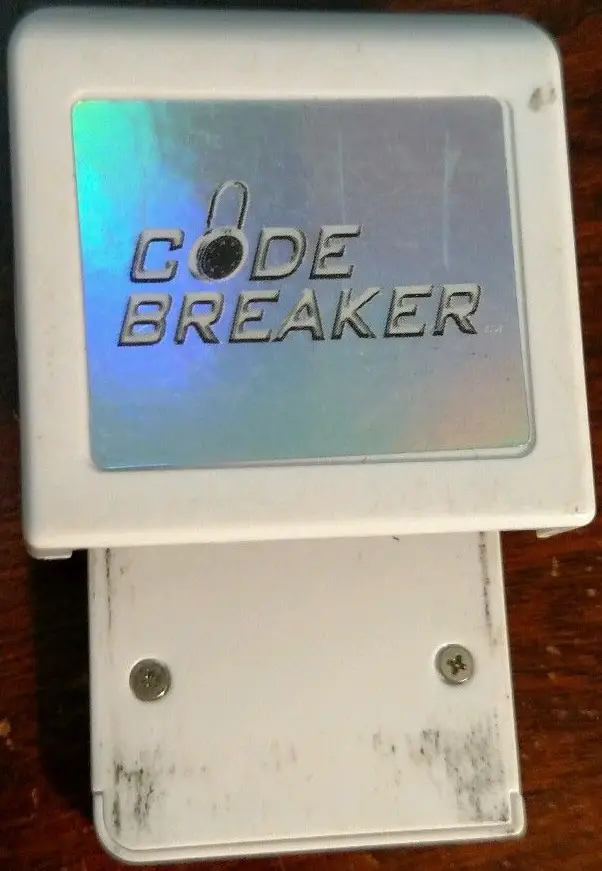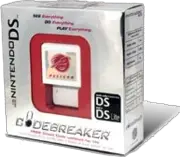# Codebreaker (Nintendo DS)

Code Breaker is a cheat device developed by Pelican Accessories, currently available for PlayStation, PlayStation 2, Dreamcast and Nintendo DS. Along with competing product Action Replay, it is one of the few currently supported video game cheat devices. The original model of the Codebreaker was identical to the Gameshark in that it would use codes to hack the game. It could also use Gameshark codes as well as its own. It was well known for having a more user friendly interface and design than the Gameshark.```   Codebreaker DS Code Types
```
```   ---------------------------------------
Auto Game Recognizer (Code Type 0x0000)
---------------------------------------
0000YYYY XXXXXXXX
XXXXXXXX = ID of the Game's Nintendo
```
```   -------------------------------------
Bit Constant Write (Code Type 0x00)
-------------------------------------
0 XXXXXXX 000000 YY
00 = Code Type Or Byte
YY = 8-bit Value
```
```   --------------------------------------
Bit Constant Write (Code Type 0x10)
--------------------------------------
XXXXXXX 1 0000 YYYY
10 = Code Type Or Byte
YYYY = 16-bit Value
```
```   --------------------------------------
Bit Constant Write (Code Type 0x20)
--------------------------------------
2 XXXXXXX YYYYYYYY
20 = Code Type Or Byte
YYYYYYYY = 32-bit Value
```
```   ---------------------------------------------
8 / Increment/Decrement Bit (Code Type 0x30)
---------------------------------------------
3 000UYYYY XXXXXXX
30 = Code Type Or Byte
U = Bit-type Write, 0 for 8 and 1 for 16-bit
YYYY = 8/16-bit Value to increment by
NOTE: YYYY is signed so it can do the increment and decrement
```
```   -------------------------------------------
Bit Increment/Decrement (Code Type 0x38)
-------------------------------------------
3 XXXXXXX YYYYYYYY
38 = Code Type Or Byte
YYYYYYYY = 8/16-bit Value to increment by
NOTE: YYYYYYYY is signed so it can do the increment and decrement
```
```   ---------------------------------------------
Serial Repeat Constant Write (Code Type 0x40)
---------------------------------------------
4 XXXXXXX TWWWZZZZ
YYYYYYYY VVVVVVVV
40 = Code Type Or Byte
T = Bit-type Write, 0 for 32-bit, 16-bit for 1 & 2 for 8
WWW = Number of times to repeat
ZZZZ = Increase Address by (Multiply by data size (1 << (2-T)))
YYYYYYYY = Start Value
VVVVVVVV = Increase Value by
Example: This code:
42010000 00050001 <--0001 * 4 = 0004 to Increase Address by
63636363 00000000
Is the same as:
22010000 63636363
22010004 63636363
22010008 63636363
2201000C 63636363
22010010 63636363
```
```   ---------------------------
Copy Bytes (Code Type 0x50)
---------------------------
5 XXXXXXX YYYYYYYY
ZZZZZZZZ 00000000
50 = Code Type Or Byte
XXXXXXX = Address to copy to
YYYYYYYY = Number of bytes to copy
ZZZZZZZZ = Address to copy from
```
```   -----------------------------
Pointer Code (Code Type 0x60)
-----------------------------
6 XXXXXXX YYYYYYYY
BCTUVVVV ZZZZZZZZ
60 = Code Type Or Byte
YYYYYYYY = 8 / 16 Bit Value
B = Bit-type Write, 0 for 8-bit, 16-bit for 1 & 2 for 32
C = Condition Enabled, 0 for disabled, 1 for enabled
T = the Condition type to check against
0 = If equal to VVVV
1 = If not equal to VVVV
2 = If lesser than VVVV
3 = If greater than VVVV
4 = If AND VVVV equal to 0000
5 = If AND VVVV not equal to 0000
6 = If AND VVVV equal to VVVV
7 = If AND VVVV not equal to VVVV
U = Bit-type Load, 0 for 8 and 1 for 16-bit
VVVV = Conditional Value to check against
```
```   -----------------------------------------------
8 / Bit Special Bitwise Write (Code Type 0x70)
-----------------------------------------------
7 00TBYYYY XXXXXXX
70 = Code Type Or Byte
T = Operation type, 0 for OR, for 1 AND 2 for XOR
B = Bit-type Write, 0 for 8 and 1 for 16-bit
YYYY = 8/16-Bit Value
```
```   ----------------------------------------------
Bit Write On Bootup-Hook 1 (Code Type 0xA0)
----------------------------------------------
AXXXXXXX YYYYYYYY
A0 = Code Type Or Byte
YYYYYYYY = Value
```
```   ----------------------------------------------
Bit Write On Bootup-Hook 2 (Code Type 0xA8)
----------------------------------------------
AXXXXXXX YYYYYYYY
A8 = Code Type Or Byte
YYYYYYYY = Value
```
```   -----------------------------------------
Bit Conditional Check (Code Type 0xD0)
-----------------------------------------
DXXXXXXX ZZTUYYYY
D0 = Code Type Or Byte
ZZ = Lines to skip, 00 means 01 by default
T = the Condition type to check against
0 = If equal to YYYY
1 = If not equal to YYYY
2 = If lesser than YYYY
3 = If greater than YYYY
4 = If AND YYYY equal to 0000
5 = If AND YYYY not equal to 0000
6 = If AND YYYY equal to YYYY
7 = If AND YYYY not equal to YYYY
U = Bit-type Load, 0, 1 for 8-bit and 16-bit for
YYYY = Conditional Value to check against
```
```   -----------------------------------
Enable Code-Hook 1 (Code Type 0xF0)
-----------------------------------
FXXXXXXX TYYYYYYY
F0 = Code Type Or Byte
T = Type of Hook to use
0 = Hook almost any 2 opcodes, LDR PC =
1 = Hook almost any BL opcode, BL function
3 = Hook almost any BX Register opcode, BX Ry
```
```   -----------------------------------
Enable Code-Hook 2 (Code Type 0xF8)
-----------------------------------
FXXXXXXX TPPPPPPP
F8 = Code Type Or Byte
XXXXXXX = Cheat Engine Hook Address
T = Type of Hook to use
0 = Hook almost any 2 opcodes, LDR PC =
1 = Hook almost any BL opcode, BL function
```   Reprinted from GSCENTRAL